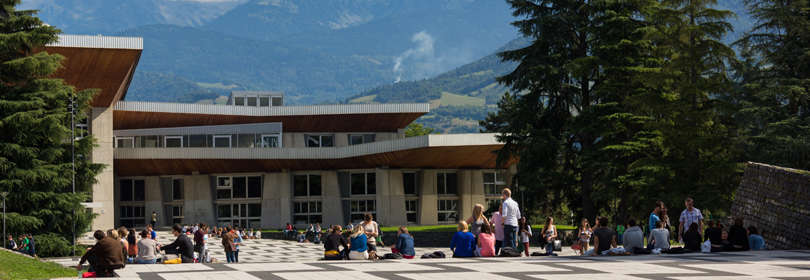Our engineering & Master degrees

School of engineering in Physics, Applied Physics, Electronics & Materials
Science# 3PMNNUM7 : Numerical methods - 4PMNXNU0

A+Augmenter la taille du texteA-Réduire la taille du texteImprimer le documentEnvoyer cette page par mail cet article Facebook Twitter Linked In
• #### Number of hours

• Lectures : 24.0
• Tutorials : 40.0
• Laboratory works : 0
• Projects : 0
• Internship : 0
ECTS : 4.0

### Goals

Acquire basic knowledge in various numerical methods applied to material science

Contact Annie FOURNIER

Content

Numerical methods for differential equations. Numerical methods for ordinary differential equations : one step methods, multi steps methods, explicit and implicit methods, prediction correction methods. Notions of precision and stability are presented. Numerical methods to solve partial differential equations : finite difference method, finite volume method, finite element method.

Prerequisites

the course of mathematic
Knowledges : derivative of one variable function and of multiple variables functions, Taylor expansion of functions ; operations between matrices, matrix and vector ; linear system of equations and vectorial operators (gradient, divergence, curl).

Tests

Semester 7 - The exam is given in english onlyWritten exam

50% note projet
50% note examen

Semester 7 - This course is given in english only